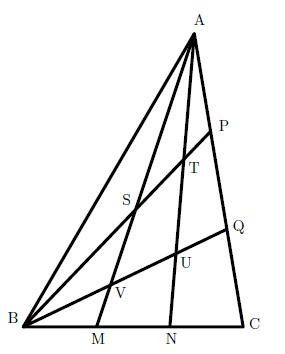# Doubly Trisected Triangle

Geometry Level 5

Triangle ABC has $\angle A = 40^{\circ}$, $\angle B = 60^{\circ}$, $\angle C = 80^{\circ}$. Points $M,N$ trisect the side $BC$ and points $P,Q$ trisect the side $AC$. The lines $AM, AN, BP, BQ$ intersect at the points $S,T,U,V$ as shown in the figure below, dividing the triangle into 9 regions. Determine the smallest possible value of $[ABC] + [STUV]$ such that both $[ABC]$ and $[STUV]$ are positive integers.Details and assumptions

$[PQRS]$ refers to the area of figure $PQRS$.

×